# Olympiad Test: Water Images - 2

## 20 Questions MCQ Test Mathematical Olympiad Class 8 | Olympiad Test: Water Images - 2

Description
Attempt Olympiad Test: Water Images - 2 | 20 questions in 40 minutes | Mock test for Class 8 preparation | Free important questions MCQ to study Mathematical Olympiad Class 8 for Class 8 Exam | Download free PDF with solutions
QUESTION: 1

Solution:
QUESTION: 2

Solution:
QUESTION: 3

### Direction: In each of the following questions, you are given a combination of alphabets and/ or numbers followed by four alternatives (a), (b), (c) and (d). Choose the alternative which most closely resembles the water-image of the given combination. 96FSH52

Solution:
QUESTION: 4

Direction: In each of the following questions, you are given a combination of alphabets and/ or numbers followed by four alternatives (a), (b), (c) and (d). Choose the alternative which most closely resembles the water-image of the given combination.

RAJ589D8

Solution:
QUESTION: 5

Direction: In each of the following questions, you are given a combination of alphabets and/ or numbers followed by four alternatives (a), (b), (c) and (d). Choose the alternative which most closely resembles the water-image of the given combination.

GR98AP76ES

Solution:
QUESTION: 6

Direction: In the following questions, choose the correct water image of the figure (X) from the given four alternatives.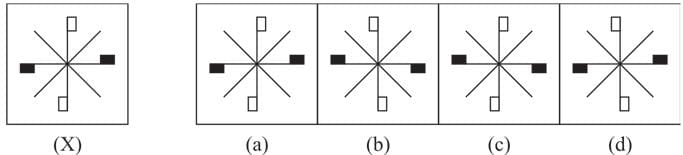Solution:
QUESTION: 7

Direction: In the following questions, choose the correct water image of the figure (X) from the given four alternatives.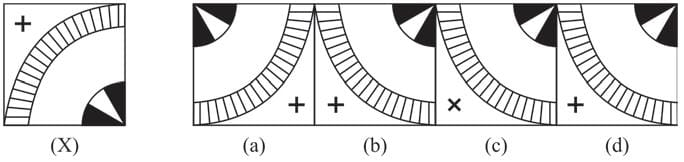Solution:
QUESTION: 8

Direction: In the following questions, choose the correct water image of the figure (X) from the given four alternatives.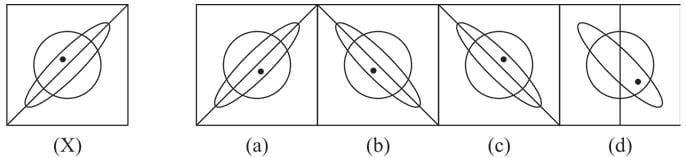Solution:
QUESTION: 9

Direction: In the following questions, choose the correct water image of the figure (X) from the given four alternatives.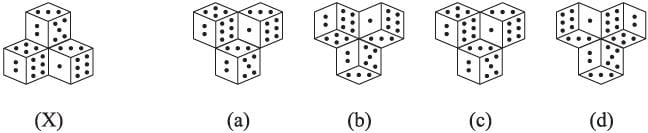Solution:
QUESTION: 10

Direction: In the following questions, choose the correct water image of the figure (X) from the given four alternatives.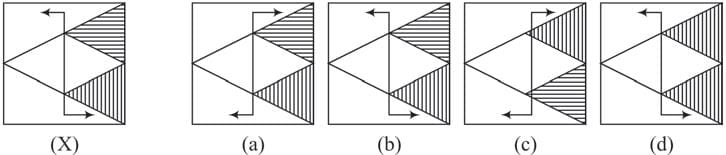Solution:
QUESTION: 11

Direction: In the following questions, choose the correct water image of the figure (X) from the given four alternatives.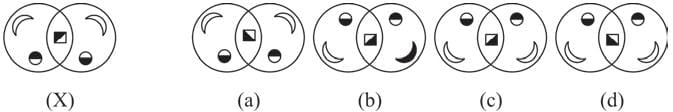Solution:
QUESTION: 12

Direction: In the following questions, choose the correct water image of the figure (X) from the given four alternatives.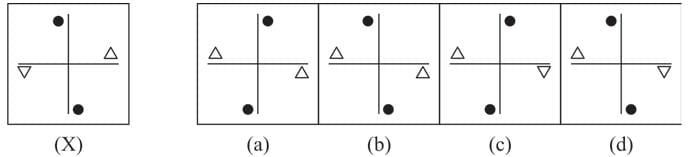Solution:
QUESTION: 13

Direction: In the following questions, choose the correct water image of the figure (X) from the given four alternatives.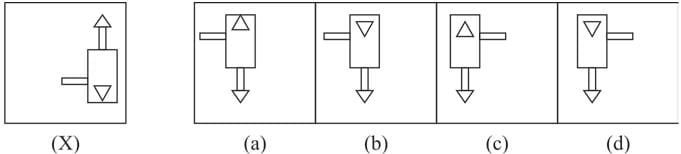Solution:
QUESTION: 14

Direction: In the following questions, choose the correct water image of the figure (X) from the given four alternatives.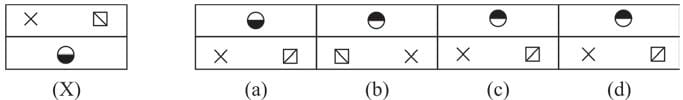Solution:
QUESTION: 15

Direction: In the following questions, choose the correct water image of the figure (X) from the given four alternatives.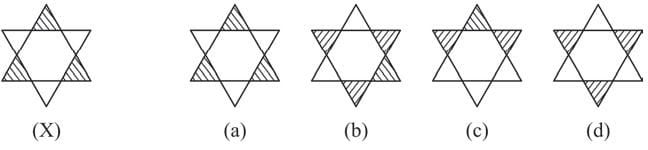Solution:
QUESTION: 16

Direction: In the following questions, choose the correct water image of the figure (X) from the given four alternatives.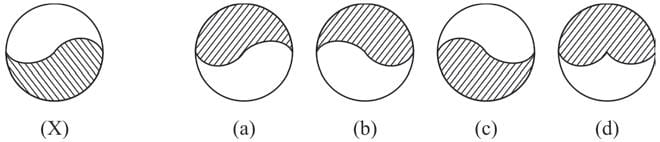Solution:
QUESTION: 17

Direction: In the following questions, choose the correct water image of the figure (X) from the given four alternatives.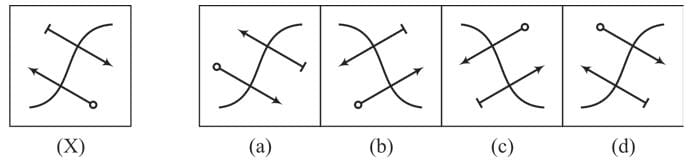Solution:
QUESTION: 18

Direction: In the following questions, choose the correct water image of the figure (X) from the given four alternatives.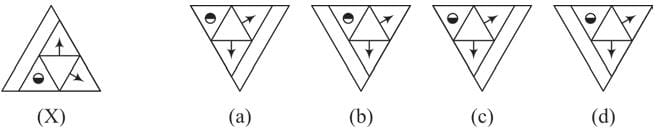Solution:
QUESTION: 19

Direction: In the following questions, choose the correct water image of the figure (X) from the given four alternatives.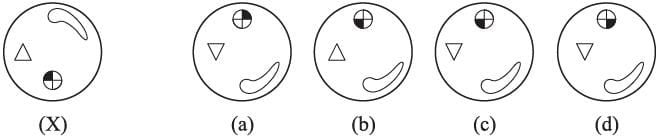Solution:
QUESTION: 20

Direction: In the following questions, choose the correct water image of the figure (X) from the given four alternatives.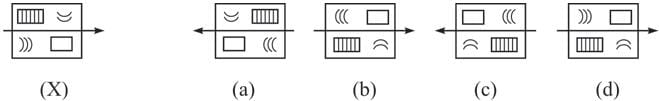Solution:Use Code STAYHOME200 and get INR 200 additional OFF Use Coupon Code